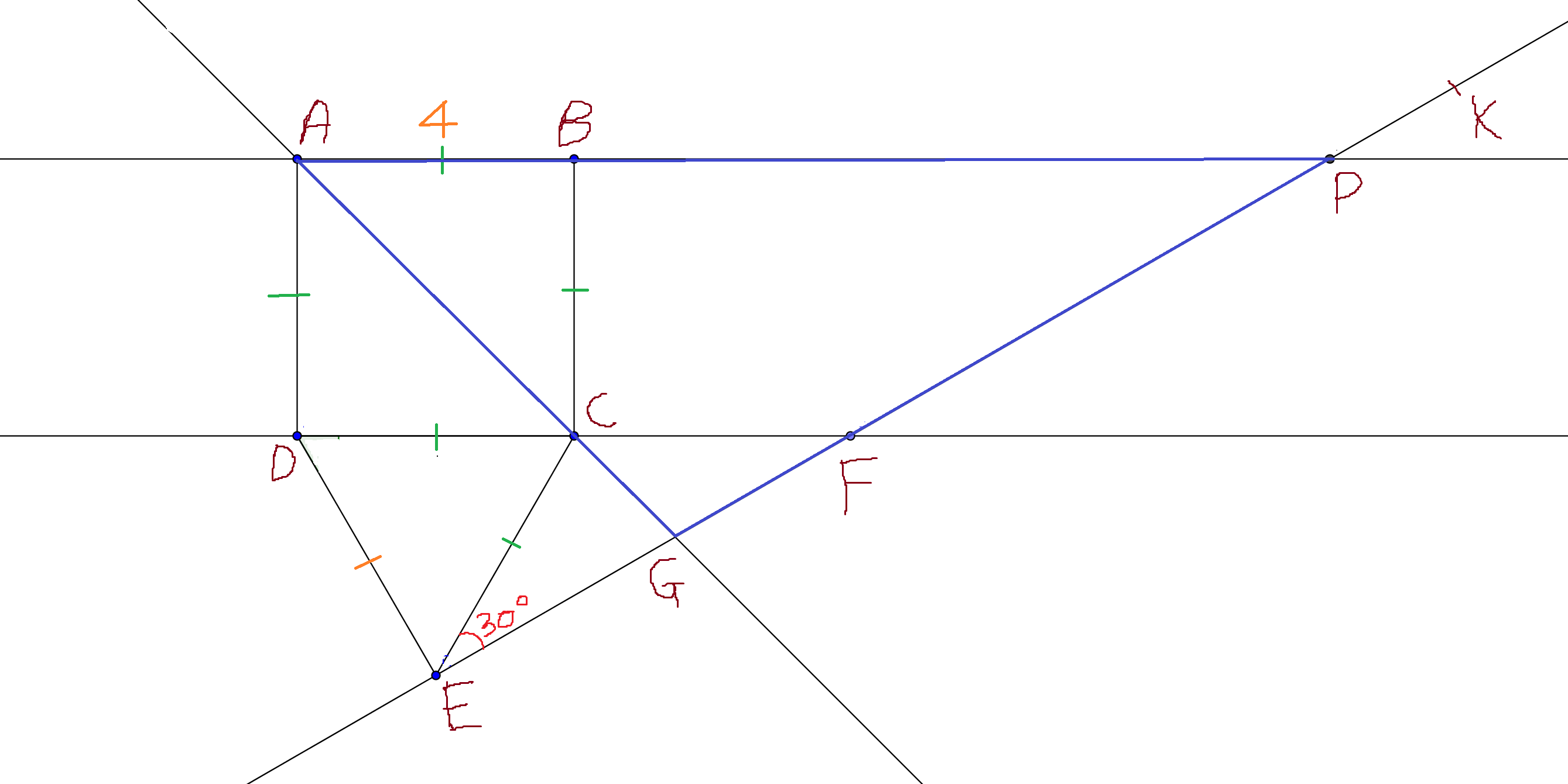Geometry Level 5Consider a square $ABCD$ with side of length $4$.

Let $E$ be a point outside $ABCD$ such that $\Delta CDE$ is equilateral.

Draw $\angle CEK = 30^\circ$ such that ray $EK$ intersects ray $AC$ at $G$, ray $DC$ at $F$, ray $AB$ at $P$.

If Area of $\Delta AGP$ can be represented as:

$a + b\sqrt{c}$ where $c$ is independent of a perfect square.

Find $a + b + c$.

×